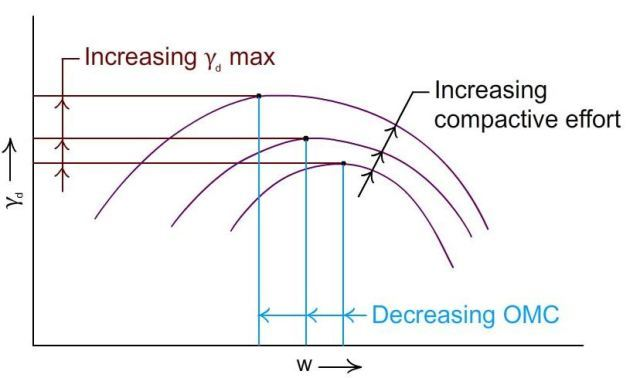# Moisture content – Dry Density Relationship

At a given input of compaction energy there exists a relationship between moisture content or water content and dry density, which is of the form shown in Fig-1.

On the dry side, the added water creates a coating of a thin film of water on the surface of the particles imparting a lubricating effect on them which enables them to move closer to each other under the influence of the compaction effort. This results in the reduction of air voids which goes on till the peak is reached at which we obtain the maximum dry density (γd max) the corresponding moisture content being called optimum moisture content (O.M.C.).Fig-1 Moisture content dry density relationship

Beyond the peak the added water starts occupying the pore space preventing the soil particles from moving into that space. As a result the dry density starts decreasing from the peak value. The corresponding moisture content is an optimum and not a maximum value unlike dry density which is a maximum value. The curve of Fig-1 is called the ‘moisture content-dry density curve’ or simply the compaction curve.

The moisture content corresponding to the ascending part of the compaction curve is marked as the dry side of optimum and that corresponding to the descending part as the wet side of optimum.

## Zero Air Voids Curve

Zero air voids correspond to a degree of saturation (S) of 100%. The relationship between γd, w and S, is as follow

ϒd = G* ϒw / [1+(G*W/S)]

Where

ϒd = Dry density of soil

G = Specific gravity of soil solids

ϒw = Unit weigh of water

W = Water content of soil

S = Degree of saturation

Substituting S = 1 (100%) we can obtain γd for various values of w and plot the result which is also shown in Fig-1 marked as ‘zero air voids curve’ or the saturation curve.

It is noted from the figure that zero air voids is a theoretical limit which can never be reached in practice, signifying thereby that it is impossible to fully expel the pore air or make the soil fully saturated in the compaction process.

The above equation can be used to plot the w-γd relationship for different values of S such as 95%, 80% etc.

## Influence of Varying the Compactive Effort

If we successively increase the compactive effort and plot the corresponding compaction curves, the results will be as shown in Fig-2.Fig-2 Effect of compactive energy on dry density and OMC

With increasing compactive effort the maximum dry density increases with decreasing OMCs, both being favorable results. These results automatically reverse sign on the decreasing side of the compactive effort.

### Article written by

Dr. Nainan P. Kurian